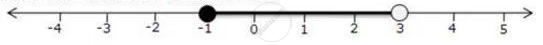Share

# For the Given Inequations Graph the Solution Set on the Real Number Line -4 < 3x - 1 < 8 - Mathematics

Course

#### Question

For the given inequations graph the solution set on the real number line

-4 < 3x - 1 < 8

#### Solution

-4  <= 3x - 1 < 8

-4 <= 3x - 1    and 3x - 1 < 8

-1 <= x and x < 3

The solution set on the real number line isIs there an error in this question or solution?